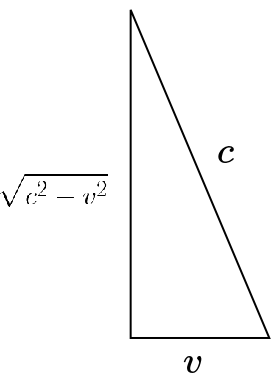文章 发现 ms@ms.lt +370 607 27 665 My work is in the Public Domain for all to share freely. 读物 书 影片 维基百科 Software Upload Understand what the key formulas for special relativity say about the Poincare group. 狭义相对论 读物 Formulas My approach is to focus on the problem, which follows from the Pythagorean theorem, that the speed of light is different for different observers. And then various resolutions are possible to this problem. But I focus on velocity.The speed of light is constant in a frame. Consider a clock where light bounces up and down, and let the clock be at rest in one frame, which is moving with velocity {$v$} in terms of another frame. For simplicity, we can consider a single bounce down. Then the speed of light as measured in the first frame appears to contract as observed from the second frame. The speed of light {$c$} is the same constant in all frames. In each frame {$f$}, the speed of light is given by {$\frac{x_f}{t_f}$}. Thus it seems that time contracts (if the distance does not) or that space contracts (if the time does not). {$x=tc$} and {$x'=t'\sqrt{c^2-v^2}$} If {$x=x'$}, then {$tc=t'\sqrt{c^2-v^2}$} and thus {$t=t'\sqrt{1-\frac{v^2}{c^2}}$}. Light clock is perpendicular to motion. If {$t=t'$}, then {$\frac{x}{c}= \frac{x'}{\sqrt{c^2-v^2}}$} and thus {$x=\frac{x'}{\sqrt{1-\frac{v^2}{c^2}}}$}. Comparing rods is parallel to motion. Rethinking the Lorentz transformation The Lorentz transformation is: {$t' = \gamma (t-\frac{vx}{c^2})$} {$x' = \gamma (x-vt)$} where {$\gamma = \frac{1}{\sqrt{1-\frac{v^2}{c^2}}}$}. Note that {$v\leq c$} implies that {$\gamma \geq 1$}. Define {$\tau = ct$}, {$\tau'=ct'$}, {$\beta=\frac{v}{c}$}. Let {$\theta$} be the angle in my right triangle which is between the leg {$\sqrt{c^2-v^2}$} and the hypotenuse {$c$}. We can have a similar right triangle with hypotenuse {$1$} and its legs will have lengths {$\alpha=\frac{1}{\gamma}$} and {$\beta$}. Thus we have {$\textrm{sec}\;\theta=\frac{1}{\alpha}=\gamma$} and {$\textrm{tan}\;\theta=\frac{\beta}{\alpha}=\beta\gamma$}. Thus we can write the Lorentz transformation as: {$\tau' = \tau\;\textrm{sec}\;\theta - x\;\textrm{tan}\;\theta$} {$x' = x\;\textrm{sec}\;\theta - \tau\;\textrm{tan}\;\theta$} Furthermore we have {$$\gamma = \textrm{sec}\;\theta = \frac{c}{\sqrt{c^2-v^2}} = \frac{c+v+c-v}{2\sqrt{c^2-v^2}}=\frac{1}{2}(\sqrt{\frac{c+v}{c-v}}+\sqrt{\frac{c-v}{c+v}})$$} {$$\beta\gamma = \textrm{tan}\;\theta = \frac{v}{\sqrt{c^2-v^2}} = \frac{c+v-c+v}{2\sqrt{c^2-v^2}}=\frac{1}{2}(\sqrt{\frac{c+v}{c-v}}-\sqrt{\frac{c-v}{c+v}})$$} {$c^2 - v^2 = 1$} We can simplify the mathematics by setting the "inferred speed of light" {$c^2 - v^2 = 1$}. (Rather than setting {$c=1$}). Then by the formulas above we get {$\textrm{sec}\;\theta = \gamma = c$} and {$\textrm{tan}\;\theta = \beta\gamma = v$}. The Lorentz transformation becomes remarkably simple: {$\tau' = \tau c - x v$} {$x' = x c - \tau v$} The transformation matrix is: {$\begin{pmatrix} c & -v \\ -v & c \end{pmatrix} \begin{pmatrix} \tau \\ x \end{pmatrix} = \begin{pmatrix} \tau' \\ x' \end{pmatrix}$} The eigenvalues and eigenvectors are: {$\begin{pmatrix} c & -v \\ -v & c \end{pmatrix} \begin{pmatrix} a \\ a \end{pmatrix} = (c - v) \begin{pmatrix} a \\ a \end{pmatrix}$} {$\begin{pmatrix} c & -v \\ -v & c \end{pmatrix} \begin{pmatrix} a \\ -a \end{pmatrix} = (c + v) \begin{pmatrix} a \\ -a \end{pmatrix}$} {$\begin{pmatrix} c & -v \\ -v & c \end{pmatrix} = \frac{1}{2a^2} \begin{pmatrix} a & -a \\ a & a \end{pmatrix} \begin{pmatrix} c-v & 0 \\ 0 & c+v \end{pmatrix} \begin{pmatrix} a & a \\ -a & a \end{pmatrix}$} Thus one eigenvector has {$x=\tau=ct$} with eigenvalue {$c - v$}. The other eigenvector has {$x=-\tau=-ct$} with eigenvalue {$c+v$}. Split complex number = hyperbolic rotation This matrix represents a split complex number {$c - jv$}. It can be thought of as a hyperbolic rotation by a hyperbolic angle. {$\begin{pmatrix} \textrm{cosh}\;a & \textrm{sinh}\;a \\ \textrm{sinh}\;a & \textrm{cosh}\;a \end{pmatrix}$} Note that the determinant of the matrix is {$\textrm{cosh}^2\;a - \textrm{sinh}^2\;a = 1$}, just as {$c^2 - v^2 = 1$}. We can write {$\textrm{cosh}\;(-a) = c = \textrm{sec}\;\theta$} and {$\textrm{sinh}\;(-a) = -v = \textrm{tan}\;\theta$}. They are different interpretations of the same triangle. {$e^a = \textrm{sec}\;\theta - \textrm{tan}\;\theta = c + v$} {$e^{-a} = \textrm{sec}\;\theta + \textrm{tan}\;\theta = c - v$} These are the two eigenvalues of the transformation matrix. These eigenvalues can be intepreted in terms of the following tangent half-angle formula: {$\textrm{tan}\;\frac{1}{2}\theta \pm \frac{\pi}{2} = \textrm{sec}\;\theta \pm \textrm{tan}\;\theta$} {$-\tau^2 + x^2$} is invariant {$\tau' = c\tau - vx$} {$x' = -v\tau + cx$} {$\tau'^2 = (c\tau - vx)^2 = c^2\tau^2 - 2cv\tau x + v^2x^2$} {$x'^2 = (-v\tau + cx)^2 = v^2\tau^2 - 2cv\tau x + c^2x^2$} {$-\tau'^2 + x^2 = (v^2-c^2)\tau^2 + (c^2-v^2)x^2 = -\tau^2 + x^2$} {$\sqrt{v^2-c^2}\tau' = i c\tau - i vx$} {$\sqrt{c^2-v^2}x' = -v\tau + cx$} {$\begin{pmatrix} x' \\ i\tau' \end{pmatrix} = \begin{pmatrix} c & -iv \\ -iv & c \end{pmatrix} \begin{pmatrix} x \\ i\tau \end{pmatrix}$} From Dr.Physics: {$s^2 = x_0^2 - x_1^2$} is invariant {$s^2 = x_0^2 - x_1^2$} {$s^2 = (ct)^2 - x_1^2$} {$s^2 = (ct)^2(1 - \frac{x_1^2}{c^2t^2})$} {$s = ct\sqrt{1 - \frac{v^2}{c^2}}$} {$\frac{s}{c} = t\sqrt{1 - \frac{v^2}{c^2}} = \tau$} Proper time. In the case of a particle, it refers to a clock traveling with that particle. {$P = m(\frac{x_0}{\tau}, \frac{x_1}{\tau}, ... )$} {$x_0 = ct$} {$p_0=\frac{mct}{\tau}$}, {$p_1=\frac{mx_1}{\tau} = \frac{mx_1}{t}\frac{t}{\tau}$} {$\frac{t}{\tau}=\frac{1}{\sqrt{1 - \frac{v^2}{c^2}}}$} {$p_0=\frac{mc}{\sqrt{1 - \frac{v^2}{c^2}}}$}, {$p_1=\frac{mv}{\sqrt{1 - \frac{v^2}{c^2}}}$} {$(1+x)^n=1 + nx + \cdots$} {$(1-\frac{v^2}{c^2})^{-\frac{1}{2}} = 1 + \frac{v^2}{2c^2} + \cdots$} {$p_1 = mv + \frac{mv^3}{2c^2}$}, {$p_0 = mc + \frac{1}{2c}mv^2 + \cdots$} {$cp_0 = mc^2 + \frac{1}{2}mv^2 + \cdots$} {$E = mc^2$} {$x_0^2 - x_1^2$} {$p_0^2 - p_1^2$} {$(mc\gamma )^2 - (mv\gamma )^2$} {$m^2\gamma^2(c^2 - v^2)$} {$m^2\gamma^2 c^2(1 - \frac{v^2}{c^2}) = m^2c^2$} {$p_0^2 - p_1^2$} {$(\frac{E}{c})^2 - p^2 = m^2c^2$} {$E^2 = c^2p^2 + m^2c^4$} {$E^2 = m^2c^4$} {$E=mc^2$} Causality Simultaneity. Same place: {$x=0$}, same time: {$t=0$}. {$t=0, x'=0$} {$t'=(t-\frac{u}{c^2}x$}\gamma = 0 because {$t=0, x=0$} {$\frac{u}{c}=\beta$} {$x_1' = \frac{x_1 - \beta x_0}{\sqrt{1-\beta^2}}$} and {$x_0' = \frac{x_0 - \beta x_1}{\sqrt{1-\beta^2}}$} {$s^2 = x_0^2 - x_1^2$} {$x_0^2 = (ct)^2$} {${x_0'}^2 - {x_1'}^2 = (x_0 - \beta x_1)^2\gamma - (x_1 - \beta x_0)^2 \gamma$} {$= \frac{x_0^2 - 2\beta x_0x_1 + \beta^2 x_1^2 - (x_1^2 - 2\beta x_0x_1 + \beta^2 x_0^2)}{1 - \beta^2}$} {$= \frac{x_0^2 + \beta^2x_1^2 - x_1^2 - \beta^2x_0}{1 - \beta^2} = x_0^2(1-\beta^2)$}
Šis puslapis paskutinį kartą keistas May 23, 2022, at 09:37 PM